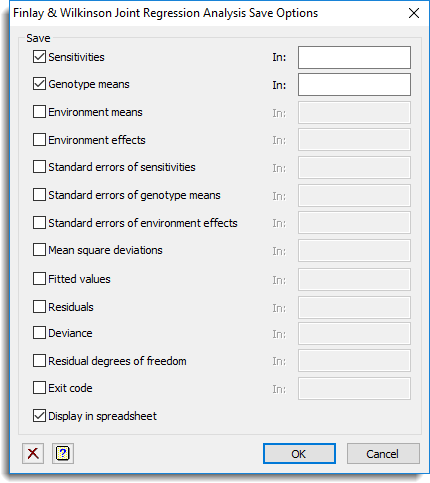1. Home
2. Finlay & Wilkinson joint regression analysis Save Options

# Finlay & Wilkinson joint regression analysis Save Options

Use this to save results from a Finlay & Wilkinson joint regression analysis in Genstat data structures.

1. After selecting the appropriate boxes, type the names for the identifiers of the data structures into the corresponding In: fields.## Save

 Sensitivities Table The estimates of sensitivities for each genotype Genotype means Table The estimates of genotype means Environment means Table The estimates of environment means Environment effects Table The estimates of environment effects Standard errors of sensitivities Table The standard errors of the sensitivities Standard errors of genotype means Table The standard errors of genotype means Standard errors of environment effects Table The standard errors of environment effects Mean square deviations Table The mean square deviations about the line fitted to each genotype Fitted values Variate The fitted values from the model Residuals Variate The residuals from the model Deviance Scalar The residual degrees of freedom from the fitted model Residual degrees of freedom Scalar The residual degrees of freedom from the model Exit code Scalar The exit status: set to 0 if the analysis converged, 1 otherwise